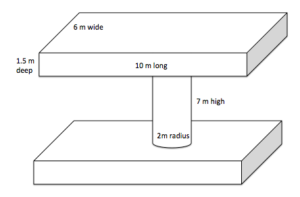## Putting It Together: Geometry

At the beginning of this module we met Tom, a carpenter at the Monterey Bay Aquarium.  Tom needs to give his supervisor two estimates, one of the volume of water they will need to have pumped into their new fish tank, and the other of the surface area of the outside of the tank, which will have a protective coating applied. The dimensions of the tank are diagrammed in the figure below.In the module we learned how to calculate the volume and surface area of some basic geometric figures, including cylinders and rectangular solids. We can answer the questions Tom has with our new knowledge of geometry.

Let’s start with the two rectangular solids that make up the top and bottom of the tank. Recall the formula for finding the volume of a rectangular solid:

$V=L\cdot W\cdot H$

We will define the length as $10m$, the width as $6m$ and the height as $1.5m$.

$V= 10m\cdot 6m\cdot 1.5m = 90m^3$

This is the volume of one of the rectangular solids, and there are two, so we can multiply by 2 to get the volume of the top and bottom sections of the tank:

$90m^3\cdot2 = 180m^3$

Now we need to find the volume of the cylinder that connects the two rectangular solids. Recall the formula for the volume of a cylinder:

$V=\pi \cdot r^2 \cdot h$

We can substitute the values for $h$ and $r$.

$V \pi \cdot (2m)^2 \cdot 7m = 28m^3$

Adding all the volumes together, we will have the final estimate that Tom can give his supervisor for the amount of water needed to fill the tank.

$180m^3+28m^3=208m^3$

Now we can tackle finding the estimate for the surface area of the tank so Tom knows how much protective coating will be needed.

The surface area of a rectangular solid is $S=2LH+2LW+2WH$, and the surface area of a cylinder with radius $r$ and height $h$, is $S=2\pi {r}^{2}+2\pi rh$. The sum of these two will give the total amount of protective coating the glass will need.

$S_{\text{rectangular solid}}+S_{\text{cylinder}}=2LH+2LW+2WH+2\pi {r}^{2}+2\pi rh$

Substituting the values from the diagram:

$2LH+2LW+2WH+2\pi {r}^{2}+2\pi rh=2(10m)(1.5m)+2(10M)(6M)+2(1.5M)(6M)+2\pi(2M)(7M)=211.98m^2$ rounded to the nearest tenth.

## Contribute!

Did you have an idea for improving this content? We’d love your input.